Courses

# Overview of Chemical Reactions and Equations (Part - 1) Class 10 Notes | EduRev

## Science Class 10

Created by: Dr Manju Sen

## Class 10 : Overview of Chemical Reactions and Equations (Part - 1) Class 10 Notes | EduRev

The document Overview of Chemical Reactions and Equations (Part - 1) Class 10 Notes | EduRev is a part of the Class 10 Course Science Class 10.
All you need of Class 10 at this link: Class 10

1. Chemical Reaction: Whenever a chemical change occurs, we say that a chemical reaction has taken place. For example, cooking of food, respiration, digestion of food, exposure of iron to humid atmosphere. Chemical change involves change of state, change of colour, evolution of a gas or change of temperature. Cooking of food, fermentation of sugar and respiration are some examples of chemical change.

Activity 1. Perform an activity to show that a chemical reaction has taken place (change in state and change in colour).

Materials required: A conical flask, 5% lead nitrate solution, 5% potassium iodide solution, test tubes.

Procedure:
(1) Take a clean 100 mL flask.
(2) Take about 10 mL (half a test tube) of lead nitrate solution in a test tube and transfer it to the flask.
(3) Then take about 10 mL of potassium iodide solution in a test tube and add it to the same flask (Fig. 1.1).
(4) Shake the flask and observe what happens.

We observe that a yellow precipitate of lead iodide is formed.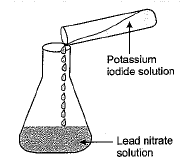Fig. 1.1 Mixing of solutions of potassium iodide and lead nitrate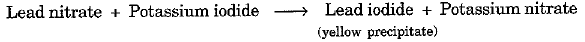Result: This activity shows that a chemical reaction has taken place. Before mixing, the two solutions were colourless. But after mixing the two solutions, we obtain a yellow insoluble substance (precipitate). Thus there is a change of state and a change of colour.

Activity 2. Perform an activity to show that a chemical reaction has taken place (evolution of a gas and change of temperature).

Materials required: Conical flask (250 mL), cork, glass tube, zinc granules, dilute sulphuric acid.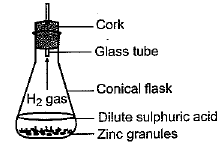Fig. 1.2 Formation of hydrogen gas by the action of dilute sulphuric acid on zincProcedure: (1) Set up the apparatus as shown in Fig. 1.2.
(2) Remove the cork and glass tube. Place some dilute sulphuric acid into it.
(3) Add a few small granules of zinc and immediately close the flask with the cork.
(4) We observe that hydrogen gas is evolved which is indicated by the bubbles.
(5) On touching the flask, we find that it is hotter now than before.

2. Chemical Equations: Description of a chemical reaction in a short form is called chemical equation. For example :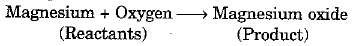Magnesium and oxygen which react are called reactants and magnesium oxide is formed after the reaction is called product. The reactants are written on the left-hand side (LHS) with a plus sign between them. Similarly the products are written on right-hand side (RHS) with a plus sign between them. The arrow head points towards the product and shows the direction of the reaction.

3. Skeletal Chemical Equation: A chemical equation written in the form of symbols and formulae is called skeletal chemical equation. Such an equation may not be balanced. For example,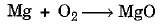In this equation, there are two oxygen atoms on the LHS while there is only one oxygen atom on RHS.

4. Balanced Chemical Equation: A chemical equation in which the number of atoms of each element on LHS and RHS is equal, is called balanced chemical equation. For example,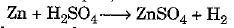is a balanced equation because the number of atoms of Zn, H, S and O is equal on the reactant (LHS) and product (RHS) sides.

5. Balancing of Chemical Equation: Let us illustrate the balancing of chemical equation by taking the example of :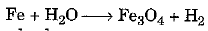The following steps are involved :
Step I. Draw boxes around each formula._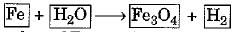Step II. We find that number of Fe and O atoms on the reactant and product sides are different. So the equation needs to be balanced.

Step III. Select the compound which has maximum number of atoms. It may be a reactant or product. In that compound, select the element which has the maximum number of atoms. Using these criteria, we select Fe304 as the compound and oxygen as the element. There are four 0 atoms on RHS and one O atom on the LHS. Hence, multiply H20 (containing O) by four to balance O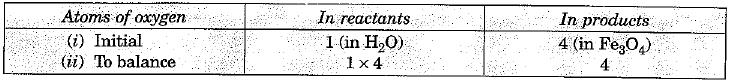The partly balanced equation becomes :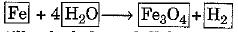Step IV. Fe and H are still to be balanced. Take either now for balancing. Let us take H. T here are eight H atoms on L H S and two H atoms on RHS. Multiply H2 by four to equalise H atoms.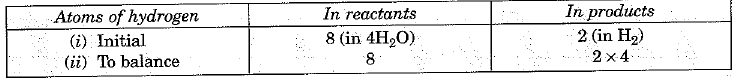The equation would become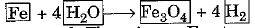Step V. Fe is left to be balanced. There is one Fe atom on LHS and three Fe atoms on RHS. Multiply Fe on LHS by three to equalise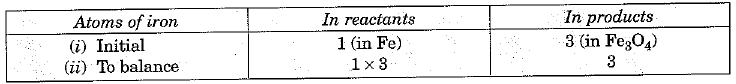We can write the equation now as :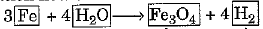Step VI. Finally we write the equation, after  removing boxes and check whether it is balanced or not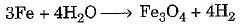This method of balancing equations is known as hit and trial method as we complete the balancing by makingtrials for different elements.

Step VII. To make the equation more informative, we write the physical states of different substances on LHS and RHS. Thus,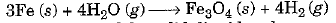Notations (s), (l) and (g) are used for solid, liquid and gaseous states respectively. In the above equation (g) after H20 means H20 is in the form of steam (gaseous ), (aq) is written for aqueous solution.

Sometimes the reaction conditions, such as temperature, pressure, catalysts, etc., for the reaction are indicated above and/or below the arrow in the equation. For example,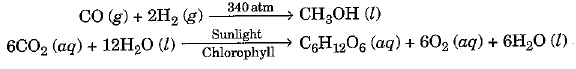TYPES OF CHEMICAL REACTIONS

Chemical reactions involve breaking and making of bonds between atoms to produce new substances.

6. Combination Reactions: A reaction in which a single product is formed from two or more reactants is called combination reaction.
For example,
(i) Burning of coal C(s) + 02 (g) → C02 (g)
(ii) Formation of water from H2 and 02. 2H2 (g) + 02 (g) → 2H20 (I)
Activity 3. Perform an activity to illustrate combination reaction .

Materials required: Beaker (500 mL ), water , calcium oxide ( quick lime).

Procedure :
(1) Take a clean 500 mL pyrex glass beaker (Fig. 1.3).
(2) Take abut 50g quick lime in it.
(4) Quick lime (calcium oxide) reacts vigorously with water to form a single product slaked lime Ca(OH)2 and a large amount of heat is evoked.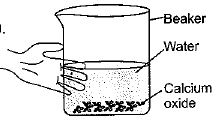F ig . 1.3 Formation of slaked lime by the reaction of calcium oxide with water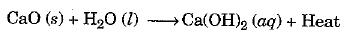Some more examples of combination reactions are : Burning of coal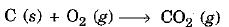Formation of water from H2 (g) and 02 (g)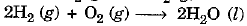,

,

,

,

,

,

,

,

,

,

,

,

,

,

,

,

,

,

,

,

,

;